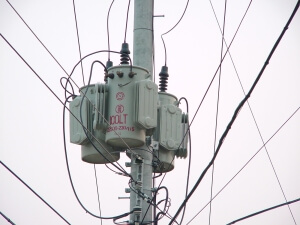# Electric Transformer

* We assume an ideal transformer where the excitation current is “0”.

## Electric transformer

A transformer is a device for simply changing the voltage of an alternating voltage (AC).
Transformers make it easy to change the voltage of an AC power source.
On the other hand, it is difficult to change the voltage that way in the DC power supply case.

The transformer consists of a primary coil and a secondary coil.
When AC power is applied to the primary coil, the magnetic field’s change causes a secondary coil’s voltage.
In general, transformers are made by putting iron cores in a coil. If you put the iron core into the coil, the magnetic field will be concentrated and react better.In many household power transformers, we can find the above structure. If you connect the home power, you can get the changed voltage.

## Voltage change of transformer

The transformer can change the voltage freely depending on the primary and secondary coils’ number of turns.
The voltage induced in the secondary coil follows the following rule:

$V_1 : V_2 = N_1 : N_2 = \frac{1}{I_1} : \frac{1}{I_2}$

V : Voltage
N : the number of turns
I : Current

A boost transformer that boosts the voltage can seem like getting energy for free.
However, as the voltage rises, the current drops, so there is no total energy gain. After all, electric power consumption is constant.

The following picture is of a transformer suspended at a utility pole.This transformer converts high voltages into low voltages. The lowered voltage is supplied to the home.
High voltage is supplied to the ‘utility pole’ because the low voltage can reduce the current more than the high voltage. When the current is reduced, the wire can be made thinner. And it can save the energy wasted through the wire.